快速傅里叶变换（FFT）算法【详解】

FFT（快速傅里叶变换）本身就是离散傅里叶变换（Discrete Fourier Transform）的快速算法，使算法复杂度由原本的O(N^2) 变为 O(NlogN)，离散傅里叶变换DFT，如同更为人熟悉的连续傅里叶变换，有如下的正、逆定义形式：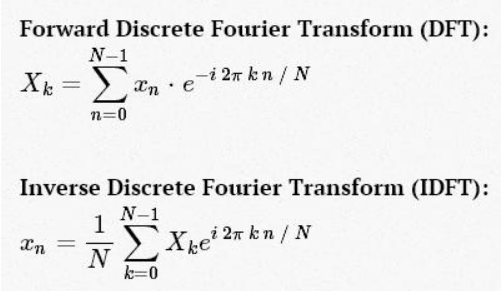xn 到 Xk 的转化就是空域到频域的转换，这个转换有助于研究信号的功率谱，和使某些问题的计算更有效率。事实上，你还可以查看一下我们即将推出的天文学和统计学的图书的第十章（这里有一些图示和python代码）。作为一个例子，你可以查看下我的文章《用python求解薛定谔方程》，是如何利用FFT将原本复杂的微分方程简化。

计算离散傅里叶变换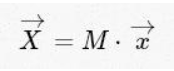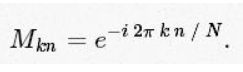1 import numpy as np
2 def DFT_slow(x):
3     """Compute the discrete Fourier Transform of the 1D array x"""
4     x = np.asarray(x, dtype=float)
5     N = x.shape
6     n = np.arange(N)
7     k = n.reshape((N, 1))
8     M = np.exp(-2j * np.pi * k * n / N)
9     return np.dot(M, x)

x = np.random.random(1024)
np.allclose(DFT_slow(x), np.fft.fft(x))

输出：

True

现在为了验证我们的算法有多慢，对比下两者的执行时间

%timeit DFT_slow(x)

%timeit np.fft.fft(x)

输出：

10 loops, best of 3: 75.4 ms per loop
10000 loops, best of 3: 25.5 µs per loop

离散傅里叶变换中的对称性

Cooley 和 Tukey 正是使用这种方法导出FFT。 首先我们来看下的值。根据上面的表达式，推出：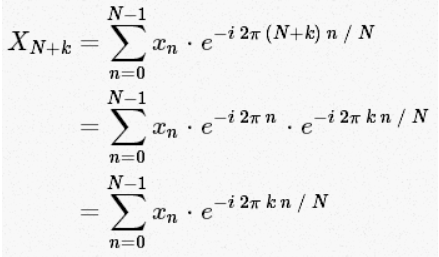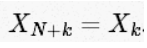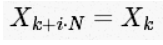DFT 到 FFT：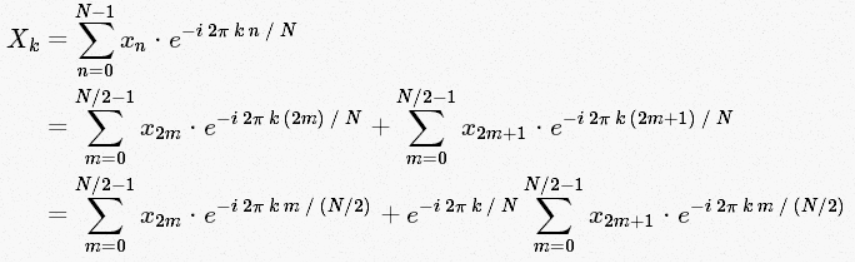1 def FFT(x):
2     """A recursive implementation of the 1D Cooley-Tukey FFT"""
3     x = np.asarray(x, dtype=float)
4     N = x.shape
5
6     if N % 2 > 0:
7         raise ValueError("size of x must be a power of 2")
8     elif N <= 32:  # this cutoff should be optimized
9         return DFT_slow(x)
10     else:
11         X_even = FFT(x[::2])
12         X_odd = FFT(x[1::2])
13         factor = np.exp(-2j * np.pi * np.arange(N) / N)
14         return np.concatenate([X_even + factor[:N / 2] * X_odd,
15                                X_even + factor[N / 2:] * X_odd])

x = np.random.random(1024)
np.allclose(FFT(x), np.fft.fft(x))

输出：

True

然后与“慢方法”的运行时间对比下：

%timeit DFT_slow(x)

%timeit FFT(x)

%timeit np.fft.fft(x)

10 loops, best of 3: 77.6 ms per loop
100 loops, best of 3: 4.07 ms per loop
10000 loops, best of 3: 24.7 µs per loop

向量化的NumPy

1 def FFT_vectorized(x):
2     """A vectorized, non-recursive version of the Cooley-Tukey FFT"""
3     x = np.asarray(x, dtype=float)
4     N = x.shape
5
6     if np.log2(N) % 1 > 0:
7         raise ValueError("size of x must be a power of 2")
8
9     # N_min here is equivalent to the stopping condition above,
10     # and should be a power of 2
11     N_min = min(N, 32)
12
13     # Perform an O[N^2] DFT on all length-N_min sub-problems at once
14     n = np.arange(N_min)
15     k = n[:, None]
16     M = np.exp(-2j * np.pi * n * k / N_min)
17     X = np.dot(M, x.reshape((N_min, -1)))
18
19     # build-up each level of the recursive calculation all at once
20     while X.shape < N:
21         X_even = X[:, :X.shape / 2]
22         X_odd = X[:, X.shape / 2:]
23         factor = np.exp(-1j * np.pi * np.arange(X.shape)
24                         / X.shape)[:, None]
25         X = np.vstack([X_even + factor * X_odd,
26                        X_even - factor * X_odd])
27
28     return X.ravel()

x = np.random.random(1024)
np.allclose(FFT_vectorized(x), np.fft.fft(x))

输出：

True

x = np.random.random(1024 * 16)
%timeit FFT(x)

%timeit FFT_vectorized(x)

%timeit np.fft.fft(x)

输出：

10 loops, best of 3: 72.8 ms per loop
100 loops, best of 3: 4.11 ms per loop
1000 loops, best of 3: 505 µs per loopposted @ 2017-05-30 00:36  Angel_Kitty  阅读(38388)  评论(5编辑  收藏  举报